Test: Combinatory- 1

# Test: Combinatory- 1

Test Description

## 20 Questions MCQ Test GATE Computer Science Engineering(CSE) 2023 Mock Test Series | Test: Combinatory- 1

Test: Combinatory- 1 for Computer Science Engineering (CSE) 2023 is part of GATE Computer Science Engineering(CSE) 2023 Mock Test Series preparation. The Test: Combinatory- 1 questions and answers have been prepared according to the Computer Science Engineering (CSE) exam syllabus.The Test: Combinatory- 1 MCQs are made for Computer Science Engineering (CSE) 2023 Exam. Find important definitions, questions, notes, meanings, examples, exercises, MCQs and online tests for Test: Combinatory- 1 below.
Solutions of Test: Combinatory- 1 questions in English are available as part of our GATE Computer Science Engineering(CSE) 2023 Mock Test Series for Computer Science Engineering (CSE) & Test: Combinatory- 1 solutions in Hindi for GATE Computer Science Engineering(CSE) 2023 Mock Test Series course. Download more important topics, notes, lectures and mock test series for Computer Science Engineering (CSE) Exam by signing up for free. Attempt Test: Combinatory- 1 | 20 questions in 60 minutes | Mock test for Computer Science Engineering (CSE) preparation | Free important questions MCQ to study GATE Computer Science Engineering(CSE) 2023 Mock Test Series for Computer Science Engineering (CSE) Exam | Download free PDF with solutions
 1 Crore+ students have signed up on EduRev. Have you?
*Answer can only contain numeric values
Test: Combinatory- 1 - Question 1

### If the ordinary generating function of a sequence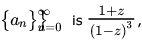then a3 - a0  is equal to ___________ .

Detailed Solution for Test: Combinatory- 1 - Question 1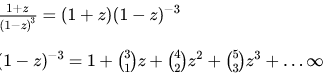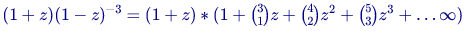a0 is the first term in the expansion of above series and  a3 is the fourth term (or) coefficient of z3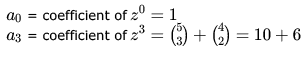⇒ a3 − a0 = 16 − 1 = 15

*Answer can only contain numeric values
Test: Combinatory- 1 - Question 2

### The coefficient of x12 in (x3 + x4 + x5 + x6 + ....) is ___________.

Detailed Solution for Test: Combinatory- 1 - Question 2

we will get x12 as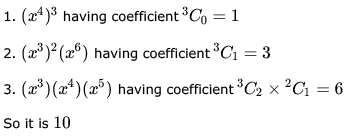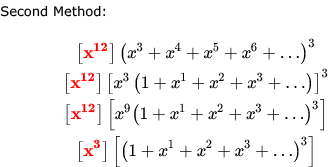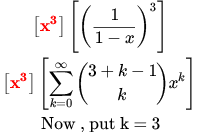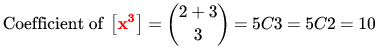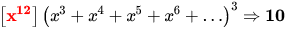Test: Combinatory- 1 - Question 3

### The coefficient of  x3 in the expansion of (1 + x)3 (2 + x2)10 is.

Detailed Solution for Test: Combinatory- 1 - Question 3

(1 + x)3 = (1 + x3 + 3x + 3x2)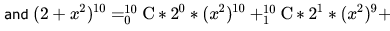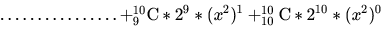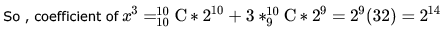As here we need to multiply last term of second expansion with first term of first coefficient (x3) and 3x with x2 in the second expansion.

Test: Combinatory- 1 - Question 4

We need to choose a team of 11 from a pool of 15 players and also select a captain. The number of different ways this can be done is

Detailed Solution for Test: Combinatory- 1 - Question 4

Number of ways selecting a captain from 15 players =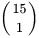Number of ways selecting remaining team members from remaining 14 players =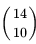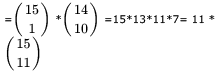Test: Combinatory- 1 - Question 5

In how many different ways can  elements be picked from a set of  elements if

(i) Repetition is not allowed and the order of picking matters?
(ii) Repetition is allowed and the order of picking does not matter?

Detailed Solution for Test: Combinatory- 1 - Question 5

(i) Repetition is not allowed and the order of picking matters =
= r arrangement with no repetition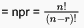(ii) Repetition is allowed and the order of picking does not matter =
= combination with unlimited repetition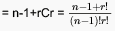Test: Combinatory- 1 - Question 6

There are  kingdoms and  champions. Each kingdom gets  champions. The number of ways in which this can be done is:

Detailed Solution for Test: Combinatory- 1 - Question 6

Option A is correct.
We have n Kingdoms as k1 , k2 , ... , kn.
Firstly we can select 2 champions from 2n champions and assign to k1 =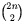ways(Say w1)

Then we can select next 2 champions and assign to k2 =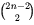ways(Say w2)
and so on..

For last kingdom , we have 2 champions left =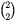ways  (say wn)

Total ways for assigning 2n champions to n kingdoms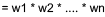=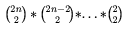= (2n)! / 2n So, Option A  (Ans) .

Test: Combinatory- 1 - Question 7

The rules for the University of Bombay five-a-side cricket competition specify that the members of each team must have birthdays in the same month. What is the minimum number of mathematics students needed to be enrolled in the department to guarantee that they can raise a team of students?

Detailed Solution for Test: Combinatory- 1 - Question 7

There are 12 months and we have to get 5 people having birthdays in the same month in order to form a team . we can apply the pigeon hole principal :

[N/12] = 5

On solving we get N=49 .

Test: Combinatory- 1 - Question 8

A  1 x 1 chessboard has one (1) square, a 2 x 2 chessboard has (5) squares. Continuing along this fashion, what is the number of squares on the (regular) 8 x 8 chessboard?

Detailed Solution for Test: Combinatory- 1 - Question 8

no of squares on chessboard of n*n is equal to sum of squares of n terms
for 8*8 chessboard
= n(n+1)(2n+1)/6
=8*9*17/6
=204

Test: Combinatory- 1 - Question 9

There is a set of 2n people:  male and  female. A good party is one with equal number of males and females (including the one where none are invited). The total number of good parties is.

Detailed Solution for Test: Combinatory- 1 - Question 9

There are n men and n women
Now we can select 1 woman from n women in nC1
With that 1 man can select  nC1 ways
So, by 1 woman and 1 man we can get   nC1 * nC1 ways...................i
Similarly , Now we can select 2 woman from n women in nC2
With that 2 man can select  nC2 ways
So, by 2 woman and 2 man we can get   nC2 * nC2 ways.........................ii .........................................................
Now, by n woman and n man we can get   nCn * nCn ways........................iii
So, by adding these equation we get
nC0.nC0 + nC1 * nC1+  nC2 * nC2 + nC3 * nC3+.......................nCn * nCn  =(2nCn)

Ans will be (E)

Test: Combinatory- 1 - Question 10

Suppose that a robot is placed on the Cartesian plane. At each step it is allowed to move either one unit up or one unit right, i.e., if it is at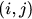then it can move to either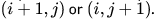Suppose that the robot is not allowed to traverse the line segment from (4,4) to (5,4). With this constraint, how many distinct paths are there for the robot to reach (10,10) starting from (0,0)?

Detailed Solution for Test: Combinatory- 1 - Question 10

Say,

r = Move Right and

u = Move Up
so using 10 combination of r and 10 combinations of u moves we get a solution.
Convert the graphical moves to text and one such solution we get =
{u, u, u, u, u, u, u, u, u, u, r, r, r, r, r, r, r, r, r, r} now all possible arrangements of them is given by =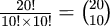now we need to discard the segment move from
(4, 4) to
(5, 5) :
to do that we first calculate how many solutions to our problem to reach (10, 10) involves that segment. We'll then subtract those solutions from the total number of solutions.
Number of solutions to reach from (0,0) to (4,4) = all possible arrangements of {r, r, r, r, u, u, u, u} =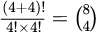definitely we take the segment (4,4) to (5,4) = 1
now, Number of solutions to reach from (5,4) to (10,10) = all possible arrangements of {r, r, r, r, r, r, u, u, u, u, u} =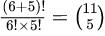*Answer can only contain numeric values
Test: Combinatory- 1 - Question 11

A palindrome is a sequence of digits which reads the same backward or forward. For example, 7447, 1001 are palindromes, but 7455, 1201 are not palindromes. How many 8 digit prime palindromes are there?

Detailed Solution for Test: Combinatory- 1 - Question 11

I think the answer should be 0, as any even digit palindrome(other than 11) cannot be prime. Even digit palindromes will always be divisible by 11(you can check the divisibility test by 11).

Test: Combinatory- 1 - Question 12

For each positive intefer n consider the set Sn defined as follows: S1 = {1}, S2 = {2, 3}, S3 = {4, 5, 6}, ... and, in general, Sn + 1 consists of  consecutinve integers the smallest of which is one more than the largest integer in Sn . Ten the sum of all the integers in S21 equals

Detailed Solution for Test: Combinatory- 1 - Question 12

Every new set Sn+1 starts after n elements from the starting element of Sn . This means that we can find the starting number of S21 using Arithmetic progression formula.
Let Sum(n) denote sum of natural numbers uptil n:
S1 starts with = 1
S2 starts with  = Sum(1) + 1= 2
S3 starts with = Sum(2) + 1 = (1+2) + 1 = 4
Similarly S21 starts with S(20) +1 =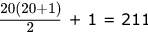Now we need to find sum of 21 consecutive natural numbers starting from 211 Using A.P. sum formula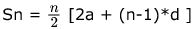where a= starting term , d= difference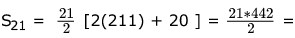4641... Option D is correct

Test: Combinatory- 1 - Question 13

For the inter-hostel six-a-side football tournament, a team of 6 players is to be chosen from 11 players consisting of 5 forwards, 4 defenders and 2 goalkeepers. The team must include at least 2 forwards, at least 2 defenders and at least 1 goalkeeper. Find the number of different ways in which the team can be chosen.

Detailed Solution for Test: Combinatory- 1 - Question 13

There are three ways to choose 6 Players.
1. 5C3*4C2*2C1=120
2. 5C2*4C2*2C2=60
3. 5C2*4C3*2C1=80
So total No of ways is 260.

Test: Combinatory- 1 - Question 14

How many substrings (of all lengths inclusive) can be formed from a character string of length n ? Assume all characters to be distinct, prove your answer.

Detailed Solution for Test: Combinatory- 1 - Question 14

Lets take an example . lets consider the given string is GATE.
so set of string of length 1 ={G,A,T,E} ; cardinality of set = 4
set of string of length 2 ={GA,AT,TE}
set of string of length 3={GAT,ATE}
set of strings of length 4 ={GATE}
and set of string of length 0 ={}
and we cant have any substring of length 5 as given string has only 4 length.
so total no of substrings are possible =0's length substring + 1lengths substrings + 2 length substrings +3 length substrings + 4 length substrings = 1+4+3+2+1
means for 1 length string to n length substrings . it will sum of the n natural no from 1 to n.
so 1+2+3+............... +n = n(n+1)/2
so total no substrings possible = 0 length strings + n(n+1)/2= 1+[n(n+1)/2]
so total no of substrings possible in n length string (All length inclusive) = 1+[n(n+1)/2]

Test: Combinatory- 1 - Question 15

How many distinct ways are there to split 50 identical coins among three people so that each person gets at least 5 coins?

Detailed Solution for Test: Combinatory- 1 - Question 15

Distinct ways are there to split 50 identical coins among three people so that each person gets at least 5 coins
x1+5+x2+5+x3+5 = 50
x1+x2+x3 = 35
Solving Non integral solution n = 35 ,r = 3
n+r-1 C r-1 = 35+3-1 C 3-1 = 37C2

Test: Combinatory- 1 - Question 16

How many disctict words can be formed by permuting the letters of the word ABRACADABRA?

Detailed Solution for Test: Combinatory- 1 - Question 16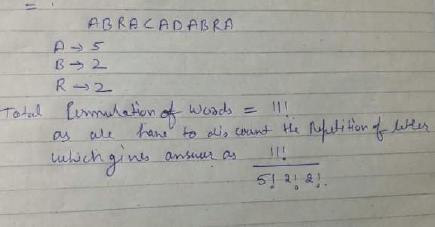Test: Combinatory- 1 - Question 17

In a tournament with 7 teams, each team plays one match with every other team. For each match, the team earns two points if it wins, one point if it ties, and no points if it loses. At the end of all matches, the teams are ordered in the descending order of their total points (the order among the teams with the same total are determined by a whimsical tournament referee). The first three teams in this ordering are then chosen to play in the next round. What is the minimum total number of points a team must earn in order to be guaranteed a place in the next round?

Detailed Solution for Test: Combinatory- 1 - Question 17

Let the 7 Teams be A,B,C,D,E,F,G and so each team plays total 6 matches.
Suppose, Team A wins over E,F,G and draws with B,C,D hence total points scored by Team A = 9 points
Now, Team B wins over E,F,G  and draws with A,C,D hence total points scored by Team B = 9 points
Similarly, happens for next two teams C and D .
Hence, Finalized scores are =>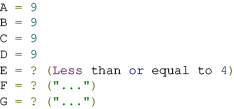Given that the order among the teams with the same total are determined by a whimsical tournament referee.
So, He/She can order the top 3 teams like ABC,ABD ,BCD ,ACD .......
But, Question says " team must earn in order to be guaranteed a place in the next round "
Hence, Not to depend on that whimsical referee, the minimum total number of points a team must earn in order to be guaranteed a place in the next round = 9 + 1 = 10 points

Test: Combinatory- 1 - Question 18

The number of permutation of {1,2,3,4,5} that keep at least one integer fixed is.

Detailed Solution for Test: Combinatory- 1 - Question 18

It is based on inclusion exclusion principle:
Total arrangements - derangements
5! - [5! - 5C1(4!) + 5C2(3!) - 5C3(2!) + 5C4(1!) - 5C5(0!)]
5C1(4!) - 5C2(3!) + 5C3(2!) - 5C4(1!) + 5C5(0!) = 120 - 60 + 20 - 5 + 1 = 76

Test: Combinatory- 1 - Question 19

A club with x members is organized into tour committees such that

(a) each member is in exactly two committees,
(b) any two committees have exactly one member in common.

Then x has

Detailed Solution for Test: Combinatory- 1 - Question 19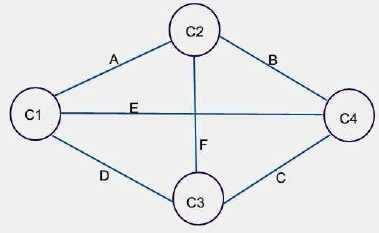Test: Combinatory- 1 - Question 20

A subset S of set of numbers {2,3,4,5,6,7,8,9,10} is said to be good if has exactly 4 elements and their gcd=1, Then number of good subset is

Detailed Solution for Test: Combinatory- 1 - Question 20

Selecting 4 numbers from 9 is 9C4 = 126
We have to subtract all the cases where gcd of all numbers is 1 and this can only happen when all number should not be even. Which is 5C4 = 5
So answer will be 126 - 5 = 121

## GATE Computer Science Engineering(CSE) 2023 Mock Test Series

150 docs|215 tests
 Use Code STAYHOME200 and get INR 200 additional OFF Use Coupon Code
Information about Test: Combinatory- 1 Page
In this test you can find the Exam questions for Test: Combinatory- 1 solved & explained in the simplest way possible. Besides giving Questions and answers for Test: Combinatory- 1, EduRev gives you an ample number of Online tests for practice

## GATE Computer Science Engineering(CSE) 2023 Mock Test Series

150 docs|215 tests